# CSI: Novelty Detection via Contrastive Learning on Distributionally Shifted Instances (NeurIPS 2020)

ALINLAB
Oct. 26, 2020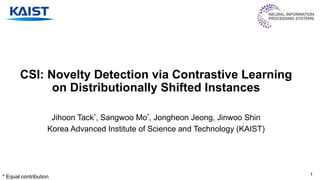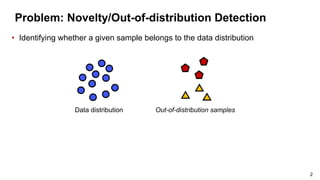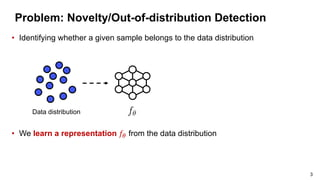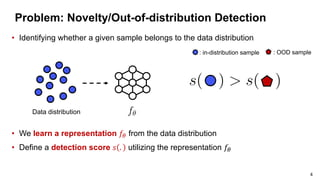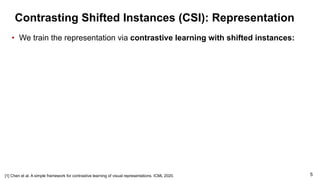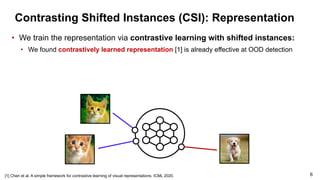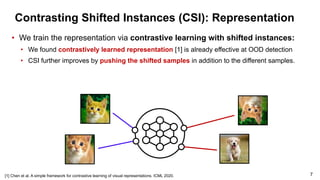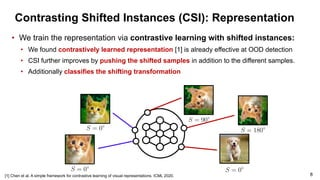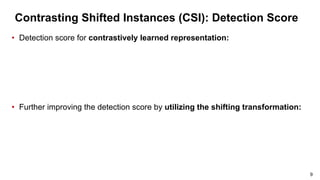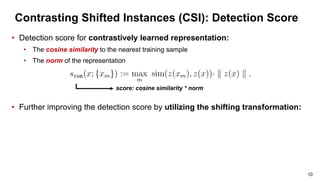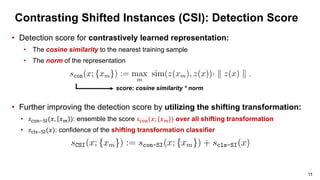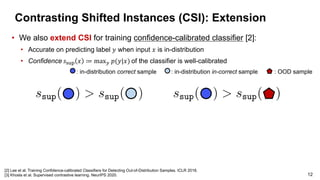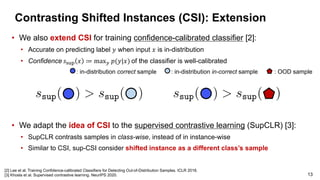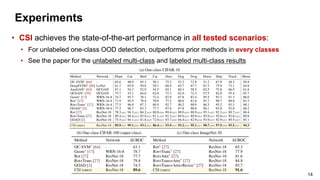1 of 15

### CSI: Novelty Detection via Contrastive Learning on Distributionally Shifted Instances (NeurIPS 2020)

1. CSI: Novelty Detection via Contrastive Learning on Distributionally Shifted Instances Jihoon Tack*, Sangwoo Mo*, Jongheon Jeong, Jinwoo Shin Korea Advanced Institute of Science and Technology (KAIST) * Equal contribution 1
2. Problem: Novelty/Out-of-distribution Detection • Identifying whether a given sample belongs to the data distribution Data distribution Out-of-distribution samples 2
3. Problem: Novelty/Out-of-distribution Detection • We learn a representation !" from the data distribution • Identifying whether a given sample belongs to the data distribution Data distribution 3
4. Problem: Novelty/Out-of-distribution Detection • We learn a representation !" from the data distribution • Define a detection score # . utilizing the representation !" • Identifying whether a given sample belongs to the data distribution Data distribution : OOD sample: in-distribution sample 4
5. Contrasting Shifted Instances (CSI): Representation 5 Chen et al. A simple framework for contrastive learning of visual representations. ICML 2020. • We train the representation via contrastive learning with shifted instances:
6. Contrasting Shifted Instances (CSI): Representation 6 Chen et al. A simple framework for contrastive learning of visual representations. ICML 2020. • We train the representation via contrastive learning with shifted instances: • We found contrastively learned representation  is already effective at OOD detection
7. Contrasting Shifted Instances (CSI): Representation 7 Chen et al. A simple framework for contrastive learning of visual representations. ICML 2020. • We train the representation via contrastive learning with shifted instances: • We found contrastively learned representation  is already effective at OOD detection • CSI further improves by pushing the shifted samples in addition to the different samples.
8. Contrasting Shifted Instances (CSI): Representation 8 Chen et al. A simple framework for contrastive learning of visual representations. ICML 2020. • We train the representation via contrastive learning with shifted instances: • We found contrastively learned representation  is already effective at OOD detection • CSI further improves by pushing the shifted samples in addition to the different samples. • Additionally classifies the shifting transformation
9. Contrasting Shifted Instances (CSI): Detection Score • Detection score for contrastively learned representation: • Further improving the detection score by utilizing the shifting transformation: 9
10. Contrasting Shifted Instances (CSI): Detection Score • Detection score for contrastively learned representation: • The cosine similarity to the nearest training sample • The norm of the representation • Further improving the detection score by utilizing the shifting transformation: 10 score: cosine similarity * norm
11. Contrasting Shifted Instances (CSI): Detection Score • Detection score for contrastively learned representation: • The cosine similarity to the nearest training sample • The norm of the representation • Further improving the detection score by utilizing the shifting transformation: • !"#\$%&'(), )+ ): ensemble the score !"#\$(); {)+}) over all shifting transformation • !"01%&'()): confidence of the shifting transformation classifier 11 score: cosine similarity * norm
12. Contrasting Shifted Instances (CSI): Extension • We also extend CSI for training confidence-calibrated classifier : • Accurate on predicting label ! when input " is in-distribution • Confidence #\$%& " ≔ max+ ,(!|") of the classifier is well-calibrated 12  Lee et al. Training Confidence-calibrated Classifiers for Detecting Out-of-Distribution Samples. ICLR 2018.  Khosla et al. Supervised contrastive learning. NeurIPS 2020. : OOD sample: in-distribution correct sample : in-distribution in-correct sample
13. Contrasting Shifted Instances (CSI): Extension • We also extend CSI for training confidence-calibrated classifier : • Accurate on predicting label ! when input " is in-distribution • Confidence #\$%& " ≔ max+ ,(!|") of the classifier is well-calibrated 13  Lee et al. Training Confidence-calibrated Classifiers for Detecting Out-of-Distribution Samples. ICLR 2018.  Khosla et al. Supervised contrastive learning. NeurIPS 2020. : OOD sample: in-distribution correct sample : in-distribution in-correct sample • We adapt the idea of CSI to the supervised contrastive learning (SupCLR) : • SupCLR contrasts samples in class-wise, instead of in instance-wise • Similar to CSI, sup-CSI consider shifted instance as a different class’s sample
14. Experiments • CSI achieves the state-of-the-art performance in all tested scenarios: • For unlabeled one-class OOD detection, outperforms prior methods in every classes • See the paper for the unlabeled multi-class and labeled multi-class results 14
15. Thank you for your attention J Paper: arxiv.org/abs/2007.08176 Code: https://github.com/alinlab/CSI 15